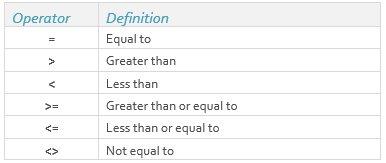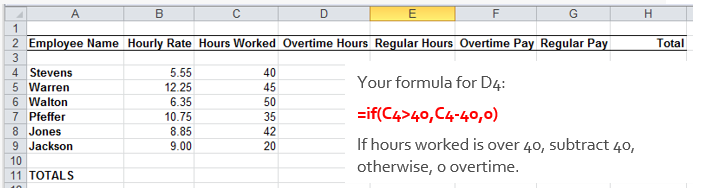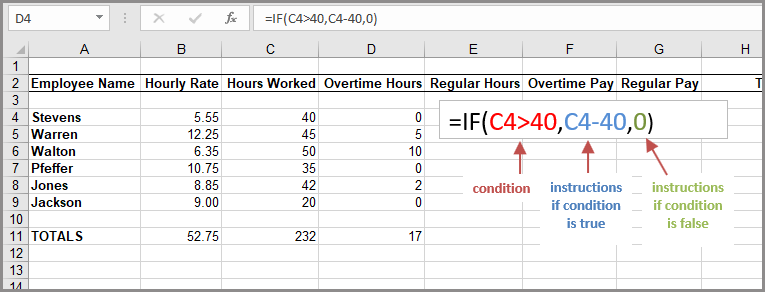Select Page

Share

You use an IF statement to ask Excel to test a condition and to return one value if the condition is met, and another value if the condition is not met.

Another way of saying it is that Excel makes a statement, and performs one calculation if the statement is true, and another calculation if the statement is false.

The format is like this:

=IF(condition, true instruction, false instruction)

A conditional test is an equation that compares two numbers, functions, formulas, labels, or logical values.

The following logical condition operators can be used in an IF() function:As an example, in the following illustration, one company considers a regular work week to be 40 hours. Hours worked over that amount will earn the employee 1½ times the hourly wage. You need a way to calculate how many overtime hours each employee worked. You can’t subtract 40 from hours worked because that would result in a negative number for those people who worked less than 40 hours.This is an excellent reason to use the IF() function. If hours worked (Column C) is over 40, subtract 40 from hours worked to come up with the number of overtime hours. If hours worked is not over 40, there are 0 overtime hours.So for line 4 in column D, the formula would be as follows:

=if(C4>40,C4–40,0)

The result depends on a comparison, in this case is one value greater than another value or not?

The following illustration shows the column filled in:So the IF() function is very versatile and can help in simple decision-making problems that involve only a few choices.